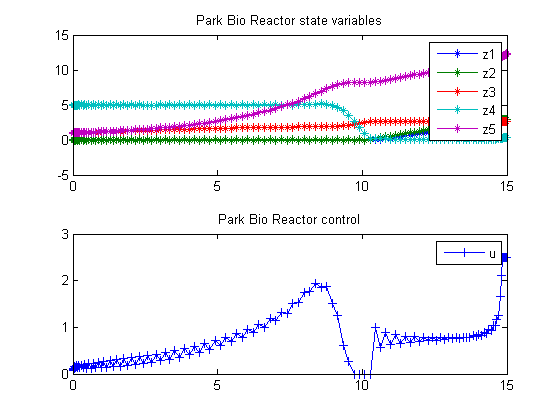82  Park-Ramirez bioreactor

Dynamic optimization of chemical and biochemical processes using restricted second-order information 2001, Eva Balsa-Canto, Julio R. Banga, Antonio A. Alonso Vassilios S. Vassiliadis

Case Study I: Park-Ramirez bioreactor

82.1  Problem description

This case study deals with the optimal production of secreted protein in a fed-batch reactor. It was originally formulated by Park and Ramirez (Park & Ramirez, 1988) and it has also been considered by other researchers (Vassiliadis, 1993; Yang & Wu, 1994; Banga, Irizarry & Seider, 1995; Luus, 1995; Tholudur & Ramirez, 1997). The objective is to maximize the secreted heterologous protein by a yeast strain in a fed-batch culture. The dynamic model accounts for host-cell growth, gene expression, and the secretion of expressed polypeptides. The mathematical statement is as follows:

Find u(t) over t in [t0,t_f] to maximize:

 J = x1(tf)*x5(tf)

subject to:

 dx1 dt
= g1*(x2x1)−
 u x5
*x1 ,
 dx2 dt
= g2*x3
 u x5
*x2 ,
 dx3 dt
= g3*x3
 u x5
*x3 ,
 dx4 dt
= −7.3*g3*x3+
 u x5
*(20−x4) ,
 dx5 dt
= u ,

with:

g1 = 4.75*
 g3 (0.12+g3)
,
g2 =
 x4 (0.1+x4)
*exp(−5*x4) ,
g3 = 21.87*
 x4 (x4+0.4)*(x4+62.5)
,

where x1 and x2 are, respectively, the concentration of the secreted protein and the total protein (l-1), x3 is the culture cell density (g l-1), x4 is the substrate concentration (g l-1), x5 is the holdup volume (l), u is the nutrient (glucose) feed rate (l h-1), and J is the mass of protein produced (in arbitrary units). The initial conditions are:

 x(t0) = [0  0  1  5  1]′

For final time t_f = 15 h, and the following constraints on the control variable:

 0 <= u <= 2

Reference: 

toms t

82.3  Solve the problem, using a successively larger number collocation points

for n=[20 40 80 120]
p = tomPhase('p', t, 0, 15, n);
setPhase(p);

tomStates z1 z2 z3 z4 z5
tomControls u

if n>20
% Interpolate an initial guess for the n collocation points
x0 = {icollocate({z1 == z1opt; z2 == z2opt
z3 == z3opt; z4 == z4opt; z5 == z5opt})
collocate(u == uopt)};
else
x0 = {};
end

% Box constraints
cbox = {icollocate({z1 <= 3
z2 <= 3; 0   <= z3 <= 4
0 <= z4 <= 10; 0.5 <= z5 <= 25})
0 <= collocate(u) <= 2.5};

% Boundary constraints
cbnd = initial({z1 == 0; z2 == 0; z3 == 1
z4 == 5; z5 == 1});

% Various constants and expressions
g3 = 21.87*z4./(z4+.4)./(z4+62.5);
g1 = 4.75*g3./(0.12+g3);
g2 = z4./(0.1+z4).*exp(-5*z4);

% ODEs and path constraints
ceq = collocate({
dot(z1) == g1.*(z2-z1)-u./z5.*z1
dot(z2) == g2.*z3-u./z5.*z2
dot(z3) == g3.*z3-u./z5.*z3
dot(z4) == -7.3*g3.*z3+u./z5.*(20-z4)
dot(z5) == u});

% Secreted protein must be less than total protein
% proteinlimit = {z1 <= z2};

% Objective
if n == 120
objective = -final(z1)*final(z5)+var(diff(collocate(u)));
else
objective = -final(z1)*final(z5);
end

82.4  Solve the problem

options = struct;
options.name = 'Park Bio Reactor';
solution = ezsolve(objective, {cbox, cbnd, ceq}, x0, options);

% Optimal z and u, to use as starting guess in the
% next iteration
z1opt = subs(z1, solution);
z2opt = subs(z2, solution);
z3opt = subs(z3, solution);
z4opt = subs(z4, solution);
z5opt = subs(z5, solution);
uopt  = subs(u, solution);
Problem type appears to be: qpcon
Starting numeric solver
===== * * * =================================================================== * * *
TOMLAB - Tomlab Optimization Inc. Development license  999001. Valid to 2011-02-05
=====================================================================================
Problem: ---  1: Park Bio Reactor               f_k     -31.891604942090215000
sum(|constr|)      0.000000000222834078
f(x_k) + sum(|constr|)    -31.891604941867381000
f(x_0)      0.000000000000000000

Solver: snopt.  EXIT=0.  INFORM=1.
SNOPT 7.2-5 NLP code
Optimality conditions satisfied

FuncEv    1 ConstrEv  102 ConJacEv  102 Iter   61 MinorIter  915
CPU time: 0.546875 sec. Elapsed time: 0.640000 sec.
Problem type appears to be: qpcon
Starting numeric solver
===== * * * =================================================================== * * *
TOMLAB - Tomlab Optimization Inc. Development license  999001. Valid to 2011-02-05
=====================================================================================
Problem: ---  1: Park Bio Reactor               f_k     -29.523702508277239000
sum(|constr|)      0.000000000532314958
f(x_k) + sum(|constr|)    -29.523702507744925000
f(x_0)    -31.891604942090005000

Solver: snopt.  EXIT=0.  INFORM=1.
SNOPT 7.2-5 NLP code
Optimality conditions satisfied

FuncEv    1 ConstrEv   75 ConJacEv   75 Iter   58 MinorIter  585
CPU time: 1.203125 sec. Elapsed time: 1.235000 sec.
Problem type appears to be: qpcon
Starting numeric solver
===== * * * =================================================================== * * *
TOMLAB - Tomlab Optimization Inc. Development license  999001. Valid to 2011-02-05
=====================================================================================
Problem: ---  1: Park Bio Reactor               f_k     -31.866762161406502000
sum(|constr|)      0.000000000725606662
f(x_k) + sum(|constr|)    -31.866762160680896000
f(x_0)    -29.523702508277385000

Solver: snopt.  EXIT=0.  INFORM=1.
SNOPT 7.2-5 NLP code
Optimality conditions satisfied

FuncEv    1 ConstrEv  137 ConJacEv  137 Iter  120 MinorIter  778
CPU time: 10.796875 sec. Elapsed time: 11.063000 sec.
Problem type appears to be: qpcon
Starting numeric solver
===== * * * =================================================================== * * *
TOMLAB - Tomlab Optimization Inc. Development license  999001. Valid to 2011-02-05
=====================================================================================
Problem: ---  1: Park Bio Reactor               f_k     -32.616144466384597000
sum(|constr|)      0.000000000325372392
f(x_k) + sum(|constr|)    -32.616144466059225000
f(x_0)    -31.477810608046273000

Solver: snopt.  EXIT=0.  INFORM=1.
SNOPT 7.2-5 NLP code
Optimality conditions satisfied

FuncEv    1 ConstrEv  134 ConJacEv  134 Iter  116 MinorIter  926
CPU time: 39.937500 sec. Elapsed time: 41.422000 sec.
end

t  = subs(collocate(t),solution);
z1 = collocate(z1opt);
z2 = collocate(z2opt);
z3 = collocate(z3opt);
z4 = collocate(z4opt);
z5 = collocate(z5opt);
u  = collocate(uopt);

82.5  Plot result

subplot(2,1,1)
plot(t,z1,'*-',t,z2,'*-',t,z3,'*-',t,z4,'*-',t,z5,'*-');
legend('z1','z2','z3','z4','z5');
title('Park Bio Reactor state variables');

subplot(2,1,2)
plot(t,u,'+-');
legend('u');
title('Park Bio Reactor control');